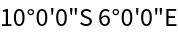#Function Repository Resource:

# GeneralizedDMSString

Convert an angle into a customizable degree-minute-second string

Contributed by: Sander Huisman
 ResourceFunction["GeneralizedDMSString"][θ] converts the angle θ in decimal degrees to a DMS string. ResourceFunction["GeneralizedDMSString"][θ,form] converts the angle θ in decimal degrees to a form string. ResourceFunction["GeneralizedDMSString"][{lat,lon}] converts latitude lat and longitude lon in decimal degrees to a DMS latitude-longitude string. ResourceFunction["GeneralizedDMSString"][{lat,lon},form] converts latitude lat and longitude lon in decimal degrees to a form latitude-longitude string. ResourceFunction["GeneralizedDMSString"][GeoPosition[{lat,lon}],form] converts a GeoPosition to a form latitude-longitude string.

## Details and Options

ResourceFunction["GeneralizedDMSString"][{θ,"NS"}] formats the angle θ as a latitude.
ResourceFunction["GeneralizedDMSString"][{θ,"EW"}] formats the angle θ as a longitude.
form can be one of the following:
 "DegreeMinuteSecond" or "DMS" or Automatic forms a string with degrees, minutes and seconds "DegreeMinute" or "DM" forms a string with degrees and minutes "Degree" or "D" forms a string with degrees
ResourceFunction["GeneralizedDMSString"] has the following options:
 "DMSSymbols" symbols to use for degree, minute and seconds "CardinalSymbols" “NESW” cardinal symbols to use "CardinalPosition" location of the cardinal symbols with respect to the numbers "LeadingDigits" number of digits before the period, padded with zeros on the left "Precision" number of digits after the period for the last number "Separators" separators to use between degrees, minutes and seconds parts and between the latitude and longitude parts
Possible forms for the options "DMSSymbols" are:
 uses ° for degrees, ' for minute and " for seconds s uses s for degrees, minutes and seconds { d } uses d for degrees and nothing for minutes and seconds { d , m } uses d for degrees, m for minutes and nothing for seconds { d , m , s } uses d for degrees, m for minutes and s for seconds uses no symbols
Possible forms for the option "CardinalSymbols" are:
 "NESW" or Automatic or True "N" for north, "E" for east, "S" for south, "W" for west "NorthEastSouthWest" "North" for north, "East" for east, "South" for south, "West" for west "NE" "N" for latitude, "E" for longitude; signs are used to indicate south and west "NorthEast" "North" for latitude, "East" for longitude; signs are used to indicate south and west { n , e } uses n for latitude, and e for longitude; signs are used to indicate south and west {{n,s},{e,w}} uses n for north, s for south, e for east, w for west uses no cardinal symbols; signs are used to indicate the hemispheres
Possible forms for the option "CardinalPosition" are:
 cardinal symbols are placed before the degrees, minutes and seconds cardinal symbols are placed after the degrees, minutes and seconds
Possible forms for the option "LeadingDigits" are:
 n uses n digits for the degrees in the latitude and the longitude { n } uses n digits for the degrees in the latitude and the longitude { n , m } uses n digits for the degrees and m for the minutes { n , m , o } uses n digits for the degrees, m for the minutes and o for the seconds uses no leading zeros uses two digits for the degree part of the latitude and three for the degree part of the longitude {{nlat,nlon},{mlat,mlon},{olat,olon}} specification for the general case for the degrees, minutes and seconds for both the latitude and the longitude
Possible forms for the option "Precision" are:
 uses six digits for the form "Degree", four digits for the form "DegreeMinute" and three for the form "DegreeMinuteSecond" n uses n digits for the last number of both the latitude and the longitude { n lat , n lon } uses nlat digits for the last number of the latitude and nlon for the last number of the longitude
Possible forms for the option "Separators" are:
 uses no separator for degree, minute and seconds and a space to separate the latitude and longitude s uses s to separate the degree, minute and seconds and the latitude and longitude parts { s } uses s to separate the degree, minute and seconds and the latitude and longitude parts { s 1 , s 2 } uses s1to separate the degree, minute and seconds and s2 for the latitude and longitude parts uses no separators
Angles in radians can be converted to degrees for use in ResourceFunction["GeneralizedDMSString"] by dividing by Degree.

## Examples

### Basic Examples

Convert an angle in a DMS string:

 In:=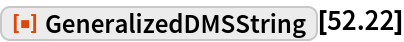Out=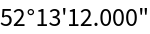Convert a latitude-longitude pair:

 In:=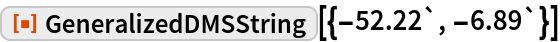Out=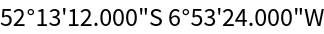Convert to a string with degrees and minutes:

 In:=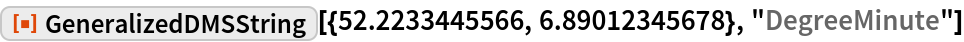Out=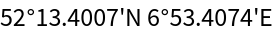Convert to a string with only degrees:

 In:=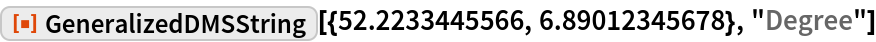Out=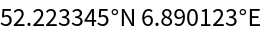### Scope

 In:=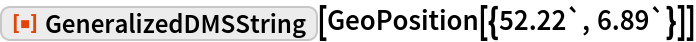Out=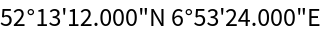Convert only a latitude into a DMS string:

 In:=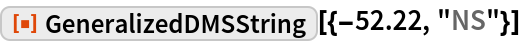Out=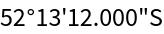Convert only a longitude into a DMS string:

 In:=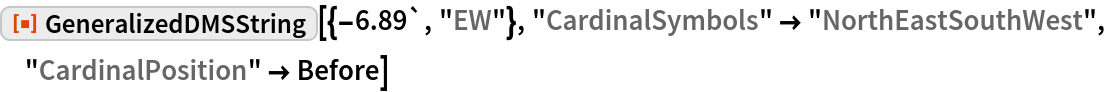Out=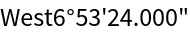### Options

#### DMSSymbols

Use different symbols for degrees, minutes and seconds:

 In:=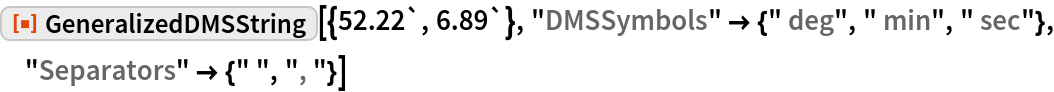Out=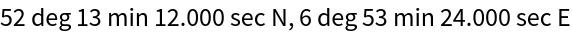Use no symbols for degrees, minutes and seconds:

 In:=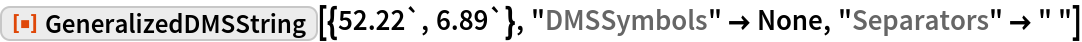Out=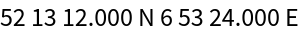Use the default symbols for degrees, minutes and seconds:

 In:=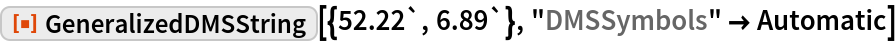Out=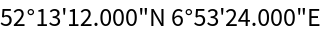#### CardinalSymbols

Use no cardinal indicators:

 In:=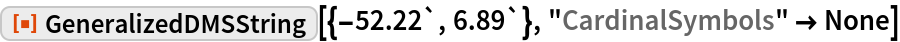Out=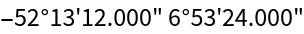Use N for the latitude and E for the longitude; signs indicate the hemisphere:

 In:=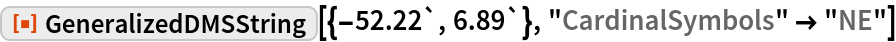Out=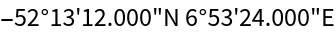Signs are removed and replaced by the proper cardinal direction:

 In:=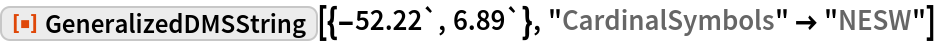Out=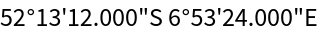Use the standard four cardinal directions written fully out:

 In:=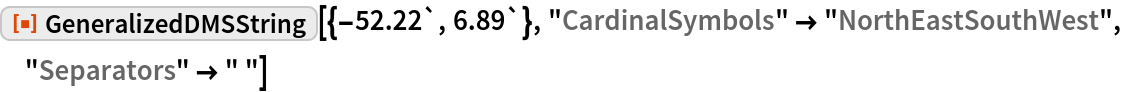Out=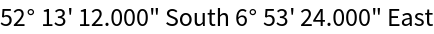Use custom cardinal directions:

 In:=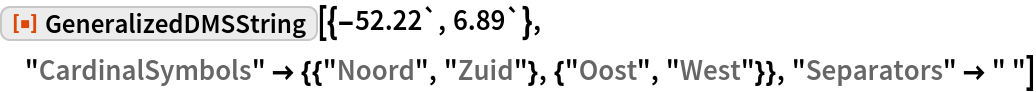Out=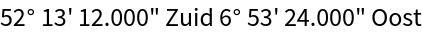Use a custom specification where the sign is retained:

 In:=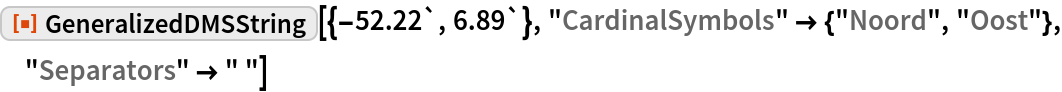Out=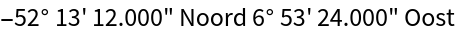#### CardinalPosition

Place the cardinal symbols before the degrees, minutes and seconds:

 In:=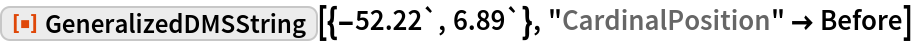Out=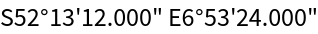Place the cardinal symbols after the degrees, minutes and seconds:

 In:=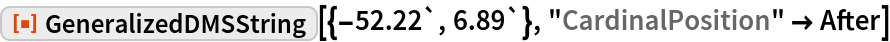Out=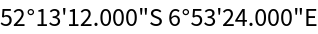Use 3 digits for degrees:

 In:=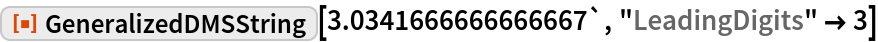Out=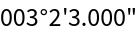Use 3 digits for degrees and 2 for minutes:

 In:=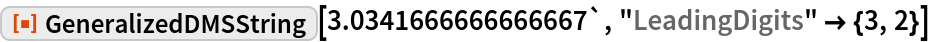Out=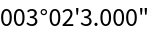Use 3 digits for degrees, 2 for minutes and 2 for seconds:

 In:=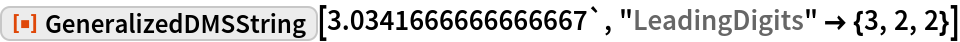Out=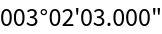Use different specifications for the latitude and longitude:

 In:=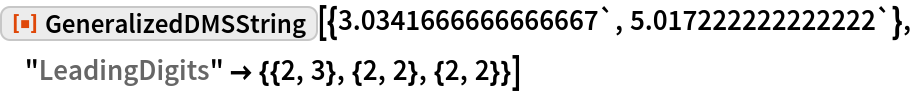Out=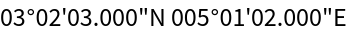#### Precision

Use 5 digits for the last number (in this case, the seconds):

 In:=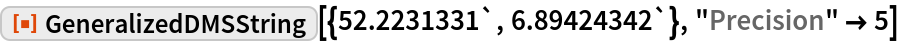Out=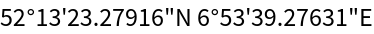Use 3 digits for the last number (in this case, the minutes):

 In:=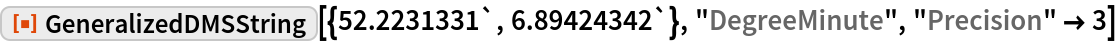Out=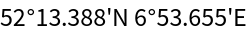#### Separators

Use underscores between degrees, minutes and seconds and between the latitude and longitude:

 In:=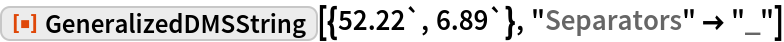Out=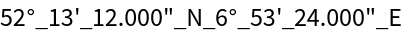Use commas between degrees, minutes and seconds, and an underscore between the latitude and longitude:

 In:=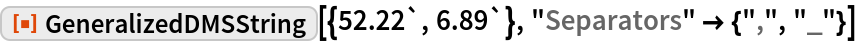Out=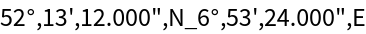Use no separators:

 In:=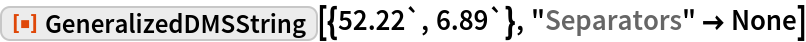Out=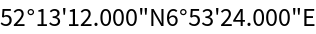### Applications

Make a function that produces a string generally used in geocaching:

 In:=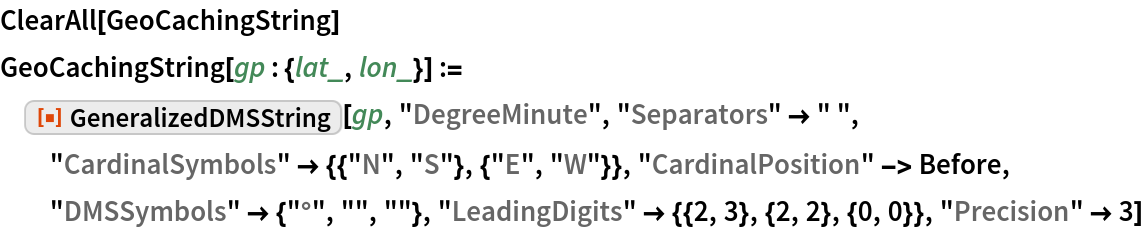Apply this function to a location:

 In:=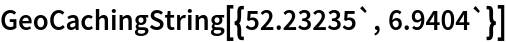Out=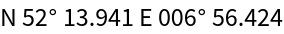### Properties and Relations

For a latitude-longitude pair, and without any options, GeneralizedDMSString gives the same output as DMSString:

 In:=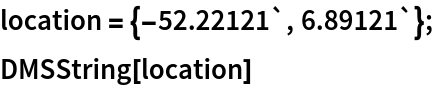Out=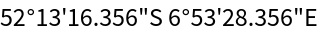In:=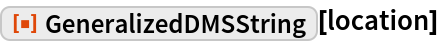Out=GeneralizedDMSString keeps the sign, as opposed to DMSString:

 In:=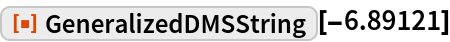Out=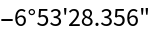In:=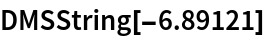Out=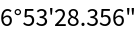### Possible Issues

Exact values are approximated using N:

 In:=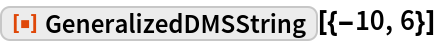Out=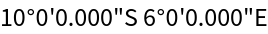Do not show the zeros behind the period:

 In:=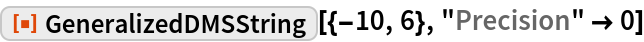Out=